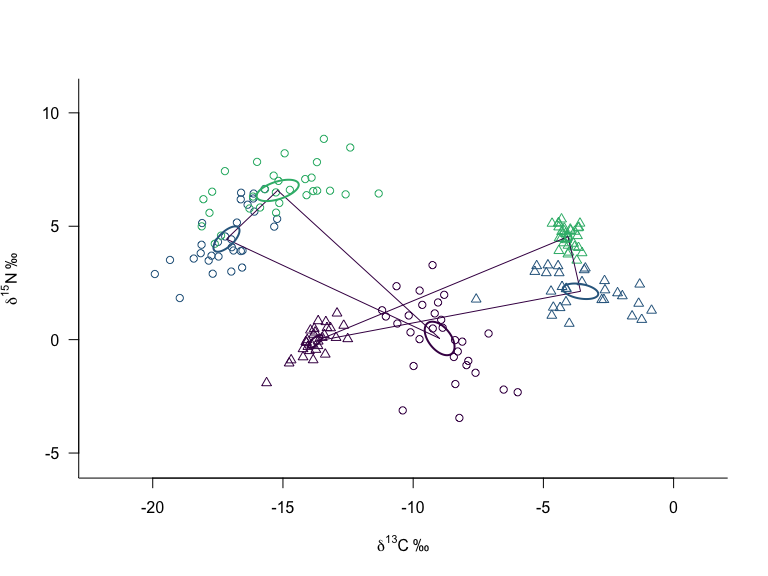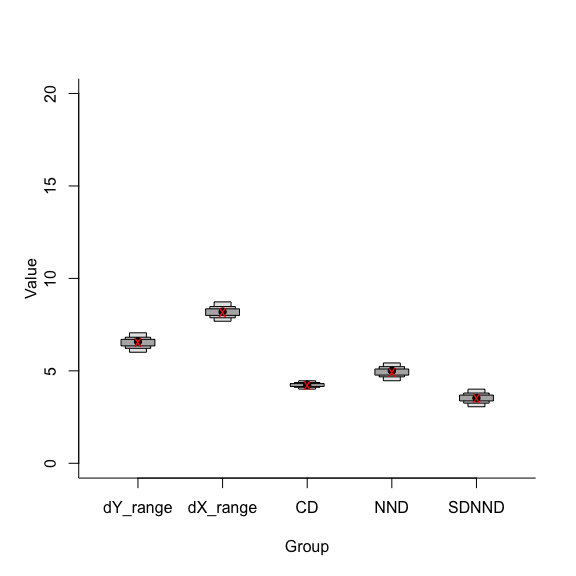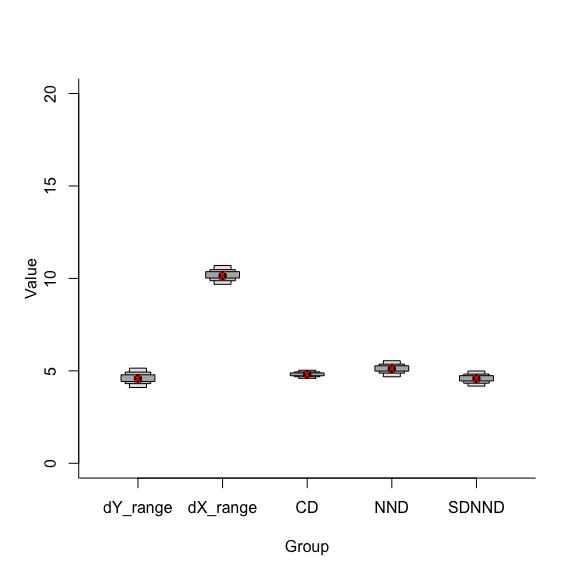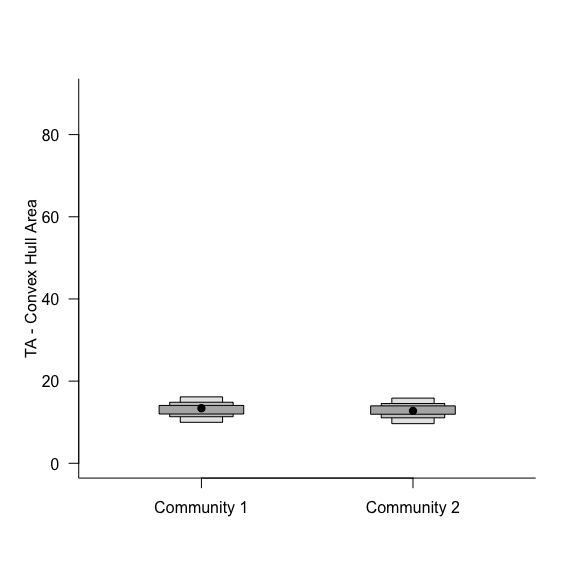# Comparing communities

#### 2023-08-22

Let us return to the original figure we explored in Introduction-to-SIBER where we had two communities side-by-side, and we wanted to ask whether they differed in how the individuals and populations within were distributed in isotope-space.

library(SIBER, quietly = TRUE,
verbose = FALSE,
logical.return = FALSE)

# Replace this line with a call to read.csv() or similar pointing to your
# own dataset.
data("demo.siber.data")
mydata <- demo.siber.data

# create the siber object
siber.example <- createSiberObject(mydata)

# Create lists of plotting arguments to be passed onwards to each
# of the three plotting functions.
community.hulls.args <- list(col = 1, lty = 1, lwd = 1)
group.ellipses.args  <- list(n = 100, p.interval = 0.95, lty = 1, lwd = 2)
group.hull.args      <- list(lty = 2, col = "grey20")

# plot the raw data
par(mfrow=c(1,1))
plotSiberObject(siber.example,
hulls = T, community.hulls.args,
ellipses = F, group.ellipses.args,
group.hulls = F, group.hull.args,
bty = "L",
iso.order = c(1,2),
xlab = expression({delta}^13*C~'\u2030'),
ylab = expression({delta}^15*N~'\u2030')
)

# add the confidence interval of the means to help locate
# the centre of each data cluster
plotGroupEllipses(siber.example, n = 100, p.interval = 0.95,
ci.mean = T, lty = 1, lwd = 2)With a convex hull drawn between the means of the populations that make up the communities, it certainly appears that Community 2 (triangles) occupies a larger portion of isotope-space than Community 1 (circles). Just as is the case when fitting ellipses to the populations within a community, there is uncertainty involved in estimating the means that describe their centroid (centre of mass). We can exploit this uncertainty to calculate a range of convex hulls that have a robust probabalistic interpretation within the Bayesian framework we use to estimate the means of the data. More than just convex hulls, we can calculate all 6 of the metrics proposed in Layman et al 2007.

• TA - the area of convex hull containing, in the case of SIBER, the means of the populations that comprise the community.
• dN_range - the distance in units between the min and max y-axis populations means which is most often d15Nitrogen in ecological studies.
• dC_range - the distance in units between the min and max x-axis population means which is most often d13Carbon in ecological studies.
• CD - the mean distance to centroid from the means
• MNND - the mean nearest neighbour distance of the means
• SDNND - the standard deviation of the nearest neighbour distance

Estimating these metrics is straight forward in SIBER once you have created the SIBER object with createSiberObject().

# Fit the Bayesian models

# options for running jags
parms <- list()
parms$n.iter <- 2 * 10^4 # number of iterations to run the model for parms$n.burnin <- 1 * 10^3 # discard the first set of values
parms$n.thin <- 10 # thin the posterior by this many parms$n.chains <- 2        # run this many chains

# define the priors
priors <- list()
priors$R <- 1 * diag(2) priors$k <- 2
priors$tau.mu <- 1.0E-3 # fit the ellipses which uses an Inverse Wishart prior # on the covariance matrix Sigma, and a vague normal prior on the # means. Fitting is via the JAGS method. ellipses.posterior <- siberMVN(siber.example, parms, priors) ## Compiling model graph ## Resolving undeclared variables ## Allocating nodes ## Graph information: ## Observed stochastic nodes: 30 ## Unobserved stochastic nodes: 3 ## Total graph size: 45 ## ## Initializing model ## ## Compiling model graph ## Resolving undeclared variables ## Allocating nodes ## Graph information: ## Observed stochastic nodes: 30 ## Unobserved stochastic nodes: 3 ## Total graph size: 45 ## ## Initializing model ## ## Compiling model graph ## Resolving undeclared variables ## Allocating nodes ## Graph information: ## Observed stochastic nodes: 30 ## Unobserved stochastic nodes: 3 ## Total graph size: 45 ## ## Initializing model ## ## Compiling model graph ## Resolving undeclared variables ## Allocating nodes ## Graph information: ## Observed stochastic nodes: 30 ## Unobserved stochastic nodes: 3 ## Total graph size: 45 ## ## Initializing model ## ## Compiling model graph ## Resolving undeclared variables ## Allocating nodes ## Graph information: ## Observed stochastic nodes: 30 ## Unobserved stochastic nodes: 3 ## Total graph size: 45 ## ## Initializing model ## ## Compiling model graph ## Resolving undeclared variables ## Allocating nodes ## Graph information: ## Observed stochastic nodes: 30 ## Unobserved stochastic nodes: 3 ## Total graph size: 45 ## ## Initializing model We can then plot out the estimated posterior distribution for each of the metrics. One of the difficulties in putting all of them on the same figure is that the convex hull (TA) tends to be much larger in magnitude (it is in units squared after all) and so it can distort the presentation of the others. I tend to plot it separately to the other metrics, at least for a first attempt. # extract the posterior means mu.post <- extractPosteriorMeans(siber.example, ellipses.posterior) # calculate the corresponding distribution of layman metrics layman.B <- bayesianLayman(mu.post) # -------------------------------------- # Visualise the first community # -------------------------------------- # drop the 3rd column of the posterior which is TA using -3. siberDensityPlot(layman.B[][ , -3], xticklabels = colnames(layman.B[][ , -3]), bty="L", ylim = c(0,20)) # add the ML estimates (if you want). Extract the correct means # from the appropriate array held within the overall array of means. comm1.layman.ml <- laymanMetrics(siber.example$ML.mu[][1,1,],
siber.example$ML.mu[][1,2,] ) # again drop the 3rd entry which relates to TA points(1:5, comm1.layman.ml$metrics[-3],
col = "red", pch = "x", lwd = 2)# --------------------------------------
# Visualise the second community
# --------------------------------------
siberDensityPlot(layman.B[][ , -3],
xticklabels = colnames(layman.B[][ , -3]),
bty="L", ylim = c(0,20))

# add the ML estimates. (if you want) Extract the correct means
# from the appropriate array held within the overall array of means.
comm2.layman.ml <- laymanMetrics(siber.example$ML.mu[][1,1,], siber.example$ML.mu[][1,2,]
)
points(1:5, comm2.layman.ml\$metrics[-3],
col = "red", pch = "x", lwd = 2)# --------------------------------------
# Alternatively, pull out TA from both and aggregate them into a
# single matrix using cbind() and plot them together on one graph.
# --------------------------------------

# go back to a 1x1 panel plot
par(mfrow=c(1,1))

# Now we only plot the TA data. We could address this as either
# layman.B[][, "TA"]
# or
# layman.B[][, 3]
siberDensityPlot(cbind(layman.B[][ , "TA"],
layman.B[][ , "TA"]),
xticklabels = c("Community 1", "Community 2"),
bty="L", ylim = c(0, 90),
las = 1,
ylab = "TA - Convex Hull Area",
xlab = "")And that pretty is pretty much that for comparing these metrics across communities, except perhaps to make a probabilistic statement about how convex hull area TA (for example) differs between Community 1 and Community 2. In this case, what is the probability that TA in Community 1 is almost the same as that in Community 2?

TA1_lt_TA2 <- sum(layman.B[][,"TA"] <
layman.B[][,"TA"]) /
length(layman.B[][,"TA"])

print(TA1_lt_TA2)
##  0.4585

And we can conclude that 45% (which is almost 50%) of the TA values estimated for Community 1 are less than Community 2. At exactly 50% the two distributions would have identical medians.